# Project: Train a Quadcopter How to Fly¶

Design an agent to fly a quadcopter, and then train it using a reinforcement learning algorithm of your choice!

Try to apply the techniques you have learnt, but also feel free to come up with innovative ideas and test them.

## Instructions¶

Take a look at the files in the directory to better understand the structure of the project.

• task.py: Define your task (environment) in this file.
• agents/: Folder containing reinforcement learning agents.
• policy_search.py: A sample agent has been provided here.
• agent.py: Develop your agent here.
• physics_sim.py: This file contains the simulator for the quadcopter. DO NOT MODIFY THIS FILE.

For this project, you will define your own task in task.py. Although we have provided a example task to get you started, you are encouraged to change it. Later in this notebook, you will learn more about how to amend this file.

You will also design a reinforcement learning agent in agent.py to complete your chosen task.

You are welcome to create any additional files to help you to organize your code. For instance, you may find it useful to define a model.py file defining any needed neural network architectures.

We provide a sample agent in the code cell below to show you how to use the sim to control the quadcopter. This agent is even simpler than the sample agent that you'll examine (in agents/policy_search.py) later in this notebook!

The agent controls the quadcopter by setting the revolutions per second on each of its four rotors. The provided agent in the Basic_Agent class below always selects a random action for each of the four rotors. These four speeds are returned by the act method as a list of four floating-point numbers.

For this project, the agent that you will implement in agents/agent.py will have a far more intelligent method for selecting actions!

In :
import random

class Basic_Agent():

def act(self):
new_thrust = random.gauss(450., 25.)
return [new_thrust + random.gauss(0., 1.) for x in range(4)]


Run the code cell below to have the agent select actions to control the quadcopter.

Feel free to change the provided values of runtime, init_pose, init_velocities, and init_angle_velocities below to change the starting conditions of the quadcopter.

The labels list below annotates statistics that are saved while running the simulation. All of this information is saved in a text file data.txt and stored in the dictionary results.

In :
%load_ext autoreload

import csv
import numpy as np

# Modify the values below to give the quadcopter a different starting position.
runtime = 5.                                     # time limit of the episode
init_pose = np.array([0., 0., 10., 0., 0., 0.])  # initial pose
init_velocities = np.array([0., 0., 0.])         # initial velocities
init_angle_velocities = np.array([0., 0., 0.])   # initial angle velocities
file_output = 'data.txt'                         # file name for saved results

# Setup
done = False
labels = ['time', 'x', 'y', 'z', 'phi', 'theta', 'psi', 'x_velocity',
'y_velocity', 'z_velocity', 'phi_velocity', 'theta_velocity',
'psi_velocity', 'rotor_speed1', 'rotor_speed2', 'rotor_speed3', 'rotor_speed4']
results = {x : [] for x in labels}

# Run the simulation, and save the results.
with open(file_output, 'w') as csvfile:
writer = csv.writer(csvfile)
writer.writerow(labels)
while True:
rotor_speeds = agent.act()
for ii in range(len(labels)):
results[labels[ii]].append(to_write[ii])
writer.writerow(to_write)
if done:
break


Run the code cell below to visualize how the position of the quadcopter evolved during the simulation.

In :
import matplotlib.pyplot as plt
%matplotlib inline

plt.plot(results['time'], results['x'], label='x')
plt.plot(results['time'], results['y'], label='y')
plt.plot(results['time'], results['z'], label='z')
plt.legend()
_ = plt.ylim()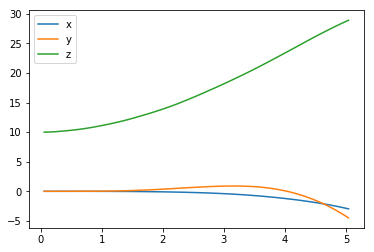The next code cell visualizes the velocity of the quadcopter.

In :
plt.plot(results['time'], results['x_velocity'], label='x_hat')
plt.plot(results['time'], results['y_velocity'], label='y_hat')
plt.plot(results['time'], results['z_velocity'], label='z_hat')
plt.legend()
_ = plt.ylim()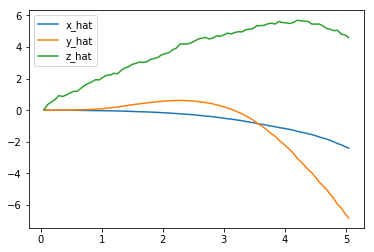Next, you can plot the Euler angles (the rotation of the quadcopter over the $x$-, $y$-, and $z$-axes),

In :
plt.plot(results['time'], results['phi'], label='phi')
plt.plot(results['time'], results['theta'], label='theta')
plt.plot(results['time'], results['psi'], label='psi')
plt.legend()
_ = plt.ylim()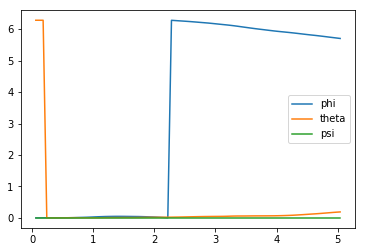before plotting the velocities (in radians per second) corresponding to each of the Euler angles.

In :
plt.plot(results['time'], results['phi_velocity'], label='phi_velocity')
plt.plot(results['time'], results['theta_velocity'], label='theta_velocity')
plt.plot(results['time'], results['psi_velocity'], label='psi_velocity')
plt.legend()
_ = plt.ylim()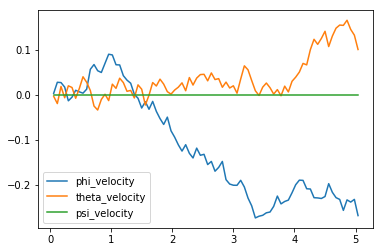Finally, you can use the code cell below to print the agent's choice of actions.

In :
plt.plot(results['time'], results['rotor_speed1'], label='Rotor 1 revolutions / second')
plt.plot(results['time'], results['rotor_speed2'], label='Rotor 2 revolutions / second')
plt.plot(results['time'], results['rotor_speed3'], label='Rotor 3 revolutions / second')
plt.plot(results['time'], results['rotor_speed4'], label='Rotor 4 revolutions / second')
plt.legend()
_ = plt.ylim()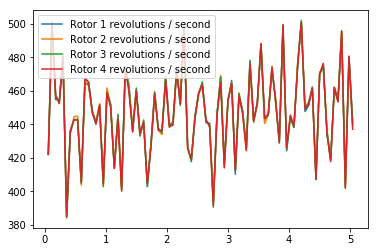When specifying a task, you will derive the environment state from the simulator. Run the code cell below to print the values of the following variables at the end of the simulation:

• task.sim.pose (the position of the quadcopter in ($x,y,z$) dimensions and the Euler angles),
• task.sim.v (the velocity of the quadcopter in ($x,y,z$) dimensions), and
• task.sim.angular_v (radians/second for each of the three Euler angles).
In :
# the pose, velocity, and angular velocity of the quadcopter at the end of the episode

[-2.97178531 -4.49976874 28.92053669  5.70411602  0.19000152  0.        ]
[-2.39786439 -6.82036716  4.60139567]
[-0.26902163  0.10099107  0.        ]


In the sample task in task.py, we use the 6-dimensional pose of the quadcopter to construct the state of the environment at each timestep. However, when amending the task for your purposes, you are welcome to expand the size of the state vector by including the velocity information. You can use any combination of the pose, velocity, and angular velocity - feel free to tinker here, and construct the state to suit your task.

A sample task has been provided for you in task.py. Open this file in a new window now.

The __init__() method is used to initialize several variables that are needed to specify the task.

• The simulator is initialized as an instance of the PhysicsSim class (from physics_sim.py).
• Inspired by the methodology in the original DDPG paper, we make use of action repeats. For each timestep of the agent, we step the simulation action_repeats timesteps. If you are not familiar with action repeats, please read the Results section in the DDPG paper.
• We set the number of elements in the state vector. For the sample task, we only work with the 6-dimensional pose information. To set the size of the state (state_size), we must take action repeats into account.
• The environment will always have a 4-dimensional action space, with one entry for each rotor (action_size=4). You can set the minimum (action_low) and maximum (action_high) values of each entry here.
• The sample task in this provided file is for the agent to reach a target position. We specify that target position as a variable.

The reset() method resets the simulator. The agent should call this method every time the episode ends. You can see an example of this in the code cell below.

The step() method is perhaps the most important. It accepts the agent's choice of action rotor_speeds, which is used to prepare the next state to pass on to the agent. Then, the reward is computed from get_reward(). The episode is considered done if the time limit has been exceeded, or the quadcopter has travelled outside of the bounds of the simulation.

In the next section, you will learn how to test the performance of an agent on this task.

## The Agent¶

The sample agent given in agents/policy_search.py uses a very simplistic linear policy to directly compute the action vector as a dot product of the state vector and a matrix of weights. Then, it randomly perturbs the parameters by adding some Gaussian noise, to produce a different policy. Based on the average reward obtained in each episode (score), it keeps track of the best set of parameters found so far, how the score is changing, and accordingly tweaks a scaling factor to widen or tighten the noise.

Run the code cell below to see how the agent performs on the sample task.

In :
import sys
import pandas as pd
from agents.policy_search import PolicySearch_Agent

num_episodes = 1000
target_pos = np.array([0., 0., 10.])

for i_episode in range(1, num_episodes+1):
state = agent.reset_episode() # start a new episode
while True:
action = agent.act(state)
agent.step(reward, done)
state = next_state
if done:
print("\rEpisode = {:4d}, score = {:7.3f} (best = {:7.3f}), noise_scale = {}".format(
i_episode, agent.score, agent.best_score, agent.noise_scale), end="")  # [debug]
break
sys.stdout.flush()

Episode = 1000, score =   1.781 (best =   2.867), noise_scale = 3.25

This agent should perform very poorly on this task. And that's where you come in!

Amend task.py to specify a task of your choosing. If you're unsure what kind of task to specify, you may like to teach your quadcopter to takeoff, hover in place, land softly, or reach a target pose.

After specifying your task, use the sample agent in agents/policy_search.py as a template to define your own agent in agents/agent.py. You can borrow whatever you need from the sample agent, including ideas on how you might modularize your code (using helper methods like act(), learn(), reset_episode(), etc.).

Note that it is highly unlikely that the first agent and task that you specify will learn well. You will likely have to tweak various hyperparameters and the reward function for your task until you arrive at reasonably good behavior.

As you develop your agent, it's important to keep an eye on how it's performing. Use the code above as inspiration to build in a mechanism to log/save the total rewards obtained in each episode to file. If the episode rewards are gradually increasing, this is an indication that your agent is learning.

In :
## TODO: Train your agent here.
import sys
from agents.agent import DDPG
import numpy as np
from random import random

# Modify the values below to give the quadcopter a different starting position.
runtime = 5.                                     # time limit of the episode
init_pose = np.array([0., 0., 0., 0., 0., 0.])  # initial pose
init_velocities = np.array([0., 0., 0.])         # initial velocities
init_angle_velocities = np.array([0., 0., 0.])   # initial angle velocities
target_pos = np.array([0., 0., 10.])
file_output = 'ddpg.txt'                         # file name for saved results
best_score = 0.0

# Setup
num_episodes = 500

labels = ['time', 'x', 'y', 'z', 'phi', 'theta', 'psi', 'x_velocity',
'y_velocity', 'z_velocity', 'phi_velocity', 'theta_velocity',
'psi_velocity', 'rotor_speed1', 'rotor_speed2', 'rotor_speed3', 'rotor_speed4', 'episode', 'total_reward']
results = {x : [] for x in labels}

# Run the simulation, and save the results.
with open(file_output, 'w') as csvfile:
writer = csv.writer(csvfile)
writer.writerow(labels)
for i_episode in range(1, num_episodes+1):
state = agent.reset_episode() # start a new episode
while True:
action = agent.act(state)
agent.step(action, reward, next_state, done)
state = next_state
# End of episode
if done:
# Update best score
if agent.total_reward > best_score:
best_score = agent.total_reward
# Logging
for ii in range(len(labels)):
results[labels[ii]].append(to_write[ii])
writer.writerow(to_write)
print("\rEpisode = {:4d}, score = {:7.3f} (best = {:7.3f})".format(
i_episode, agent.total_reward, best_score), end="")  # [debug]
break
sys.stdout.flush()

Episode =  500, score = 229.423 (best = 241.552)

## Plot the Rewards¶

Once you are satisfied with your performance, plot the episode rewards, either from a single run, or averaged over multiple runs.

In :
## TODO: Plot the rewards.
%matplotlib inline
import matplotlib.pyplot as plt

plt.figure(figsize=(12, 5))
plt.plot(results['episode'], results['total_reward'])
plt.xlabel('episode')
plt.ylabel('rewards')
plt.show()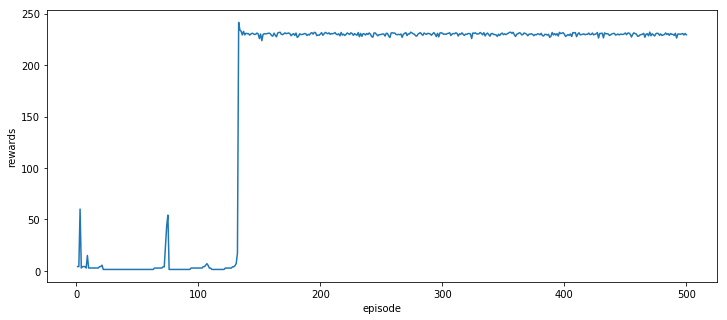In :
# Average of last 10 episodes
np.mean(results['total_reward'][-10:])

Out:
229.7969701951196
In :
plt.plot(results['episode'], results['x'], label='x')
plt.plot(results['episode'], results['y'], label='y')
plt.plot(results['episode'], results['z'], label='z')
plt.legend()
plt.show()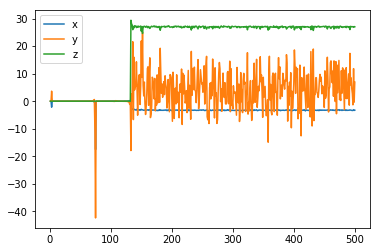In :
plt.plot(results['episode'], results['x_velocity'], label='x_hat')
plt.plot(results['episode'], results['y_velocity'], label='y_hat')
plt.plot(results['episode'], results['z_velocity'], label='z_hat')
plt.legend()
_ = plt.ylim()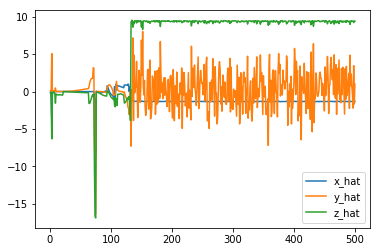## Reflections¶

Question 1: Describe the task that you specified in task.py. How did you design the reward function?

• I started with the default task provided by udacity and realized that it needs a lot of tweaks there in get reward function.
• My intuition told me to just add only the positive reward to my quadcopter. Firstly, I focused on only z position and velocity by feeding the rewards based on the current position/velocity, I numerously iterated finding a suitable parameters there. However, my quadcopter didn't response quite well. So, I added some rewards on runtime and boundaries when the quadcopter stayed in my boundaries. The problem is that it didn't work so I decided to remove them.
• I then started to implement the punishment to my lovely quadcopter by penalizing x, y position, euler angles, and all of x,y velocity using the norms of current positions and ideal positions. The problem with my negative rewards were that they limited the way quadcopter fly and made quadcopter crash so fast.
• I think adding too many negative rewards and many constraints/conditions do not work well because it will make quadcopters fail to learn how to fly properly and crash in just 1 second after taking off - think of it like we remove all of our arms/ legs and still want to walk/ run , it doesn't make sense at all.
• Thus, I removed all of my negative rewards but x,y position with small weight. Besides, I added a very minimal positve reward based on current z position. This makes my agent free to learn in whatever she wants.
• After keep it stupid simple, I normalize the reward with np.tanh function so that my quadcopter is not effected much with large/small values.

Question 2: Discuss your agent briefly, using the following questions as a guide:

• What learning algorithm(s) did you try? What worked best for you?
• What was your final choice of hyperparameters (such as $\alpha$, $\gamma$, $\epsilon$, etc.)?
• What neural network architecture did you use (if any)? Specify layers, sizes, activation functions, etc.

• I use DDPG actor-critic method for this project. Actor-critic is an interesting algorithm to study because of it combines both value based and policy based method together making it has better convergence to local/global optima and also more suitable for continous action space problem. Another reason is that udacity provided a lot of bootstrap code to me so I would go with this algorithm.
• Because this is machine learning course and I have a lot of experience with tuning parameters based on previous projects, I immedietely jumped into searching for best hyperparameters and played with a lot of neural network architectures; however, I was quite dissapointed and realized that the most important part of this project is to design your own reward function. The parameters and architectures udacity provided are fairly optimal and work very good in most case. I spend a significant time and decide to stick and tweak just a little of the default parameters.
• I use 3 hidden layers for actor model with 32 or 64 nodes per layers. Adding more layers or some regularizations like dropout makes nn harder to converge and learn. I often see nn did not learn or fail miserably to learn because of my complex model so I decide to use much simple architecture and focus mostly on design reward function. The same story went on critic model and I also decided to spend most of my time on tweaking noise parameters, and designing reward function.
• I use 0.99 discount factors and 0.01 tau. I try to reduce discount factors less than this but the agent didn't response quite well; maybe because it focuses on short goals and crash frequently. Adding more tau value might skip the local optima.
• After various searchs for the noise parameters, I decided to just use the default one (mu=0.0 theta=0.15 sigma =0.2) and spend most of my energy with designing reward function. Tweaking mu, sigma, theta makes agent love to explore and not going to exploit. It is about exploration vs explotation. Perhaps, I have spent just a little time on this but personally, I think I should focus more on reward function.

Question 3: Using the episode rewards plot, discuss how the agent learned over time.

• Was it an easy task to learn or hard?
• Was there a gradual learning curve, or an aha moment?
• How good was the final performance of the agent? (e.g. mean rewards over the last 10 episodes)

• Reinforcement learning is never easy, this project too. I found out that finding the proper reward function is super diffcult and it sucks a lot of my time. After finding the reward function, the rest seems easier. Although my agent can act and fly better than the previous model using policy search, it doesn't mean this is an easy task for sure.
• The aha moment for my agent is existed. The learning curve always be like this: bad > bad > bad > suddenly jump to the better result > bad > plateau for a long time. . . > aha moment! > consistency.
• According to my plot, the aha moment came in around 120 episodes. After that episode, it seems my agent know how to fly and learn how to keep consistent on that. This is very great because many of my previous attempts failed to remember the success before dropping dramatically and never got back to the best score ever.
• This plot shows that this agent is very stable and know how to hit the target already after reaching 120 episode.
• The final performance is good and consistent with an average reward of 229 for last 10 episodes.

Question 4: Briefly summarize your experience working on this project. You can use the following prompts for ideas.

• What was the hardest part of the project? (e.g. getting started, plotting, specifying the task, etc.)
• Did you find anything interesting in how the quadcopter or your agent behaved?